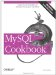# Counting Missing Values

13.5.1 Problem

A set of observations is incomplete. You want to find out how much so.

13.5.2 Solution

Count the number of NULL values in the set.

13.5.3 Discussion

Values can be missing from a set of observations for any number of reasons: A test may not yet have been administered, something may have gone wrong during the test that requires invalidating the observation, and so forth. You can represent such observations in a dataset as NULL values to signify that they're missing or otherwise invalid, then use summary queries to characterize the completeness of the dataset.

If a table t contains values to be summarized along a single dimension, a simple summary will do to characterize the missing values. Suppose t looks like this:

```mysql> SELECT subject, score FROM t ORDER BY subject;
+---------+-------+
| subject | score |
+---------+-------+
| 1 | 38 |
| 2 | NULL |
| 3 | 47 |
| 4 | NULL |
| 5 | 37 |
| 6 | 45 |
| 7 | 54 |
| 8 | NULL |
| 9 | 40 |
| 10 | 49 |
+---------+-------+```

COUNT(*) counts the total number of rows and COUNT(score) counts only the number of non-missing scores. The difference between the two is the number of missing scores, and that difference in relation to the total provides the percentage of missing scores. These calculations are expressed as follows:

```mysql> SELECT COUNT(*) AS 'n (total)',
-> COUNT(score) AS 'n (non-missing)',
-> COUNT(*) - COUNT(score) AS 'n (missing)',
-> ((COUNT(*) - COUNT(score)) * 100) / COUNT(*) AS '% missing'
-> FROM t;
+-----------+-----------------+-------------+-----------+
| n (total) | n (non-missing) | n (missing) | % missing |
+-----------+-----------------+-------------+-----------+
| 10 | 7 | 3 | 30.00 |
+-----------+-----------------+-------------+-----------+```

As an alternative to counting NULL values as the difference between counts, you can count them directly using SUM(ISNULL(score)). The ISNULL( ) function returns 1 if its argument is NULL, zero otherwise:

```mysql> SELECT COUNT(*) AS 'n (total)',
-> COUNT(score) AS 'n (non-missing)',
-> SUM(ISNULL(score)) AS 'n (missing)',
-> (SUM(ISNULL(score)) * 100) / COUNT(*) AS '% missing'
-> FROM t;
+-----------+-----------------+-------------+-----------+
| n (total) | n (non-missing) | n (missing) | % missing |
+-----------+-----------------+-------------+-----------+
| 10 | 7 | 3 | 30.00 |
+-----------+-----------------+-------------+-----------+```

If values are arranged in groups, occurrences of NULL values can be assessed on a per-group basis. Suppose t contains scores for subjects that are distributed among conditions for two factors A and B, each of which has two levels:

```mysql> SELECT subject, A, B, score FROM t ORDER BY subject;
+---------+------+------+-------+
| subject | A | B | score |
+---------+------+------+-------+
| 1 | 1 | 1 | 18 |
| 2 | 1 | 1 | NULL |
| 3 | 1 | 1 | 23 |
| 4 | 1 | 1 | 24 |
| 5 | 1 | 2 | 17 |
| 6 | 1 | 2 | 23 |
| 7 | 1 | 2 | 29 |
| 8 | 1 | 2 | 32 |
| 9 | 2 | 1 | 17 |
| 10 | 2 | 1 | NULL |
| 11 | 2 | 1 | NULL |
| 12 | 2 | 1 | 25 |
| 13 | 2 | 2 | NULL |
| 14 | 2 | 2 | 33 |
| 15 | 2 | 2 | 34 |
| 16 | 2 | 2 | 37 |
+---------+------+------+-------+```

In this case, the query uses a GROUP BY clause to produce a summary for each combination of conditions:

```mysql> SELECT A, B, COUNT(*) AS 'n (total)',
-> COUNT(score) AS 'n (non-missing)',
-> COUNT(*) - COUNT(score) AS 'n (missing)',
-> ((COUNT(*) - COUNT(score)) * 100) / COUNT(*) AS '% missing'
-> FROM t
-> GROUP BY A, B;
+------+------+-----------+-----------------+-------------+-----------+
| A | B | n (total) | n (non-missing) | n (missing) | % missing |
+------+------+-----------+-----------------+-------------+-----------+
| 1 | 1 | 4 | 3 | 1 | 25.00 |
| 1 | 2 | 4 | 4 | 0 | 0.00 |
| 2 | 1 | 4 | 2 | 2 | 50.00 |
| 2 | 2 | 4 | 3 | 1 | 25.00 |
+------+------+-----------+-----------------+-------------+-----------+```MySQL Cookbook
ISBN: 059652708X
EAN: 2147483647
Year: 2005
Pages: 412
Authors: Paul DuBois